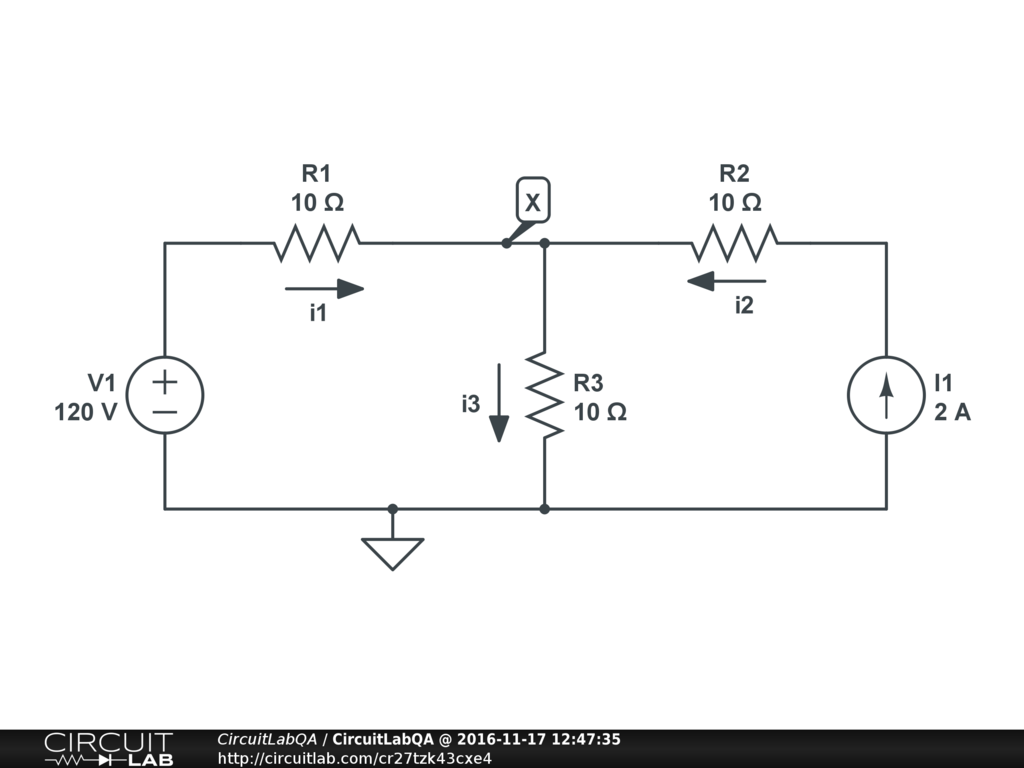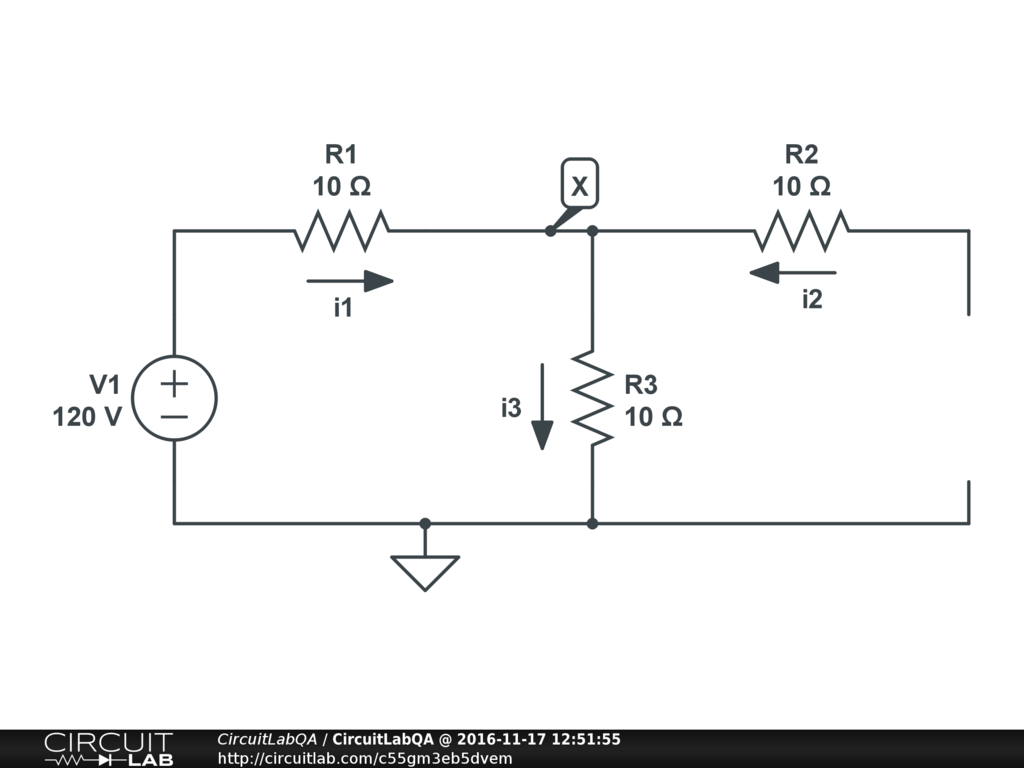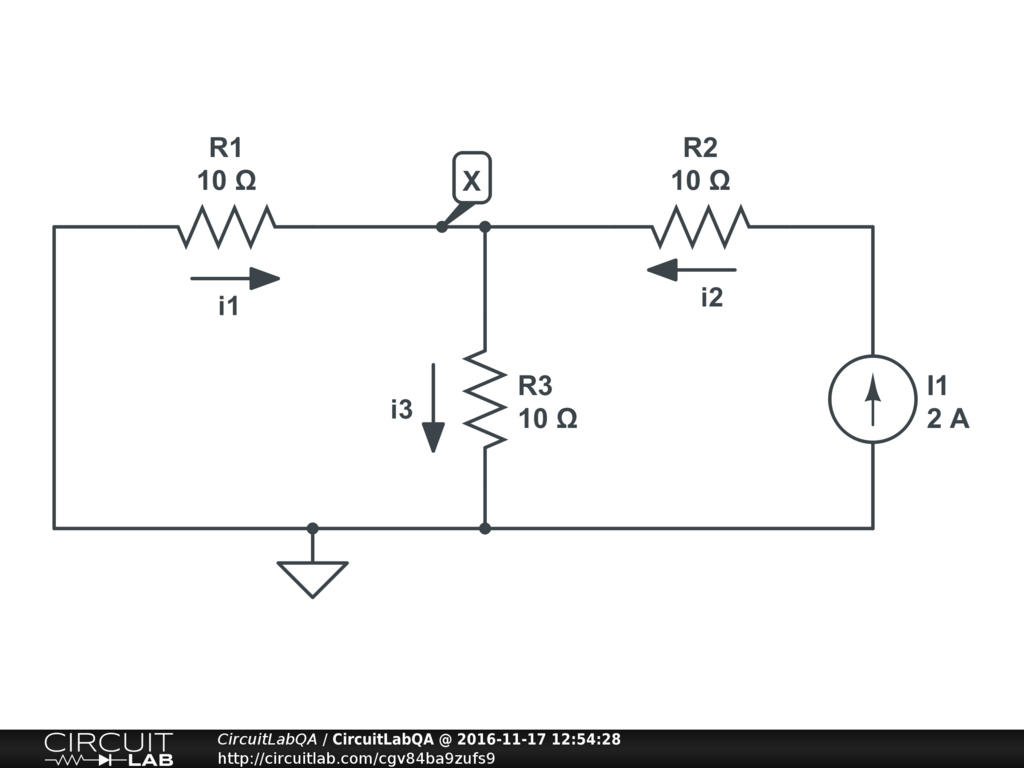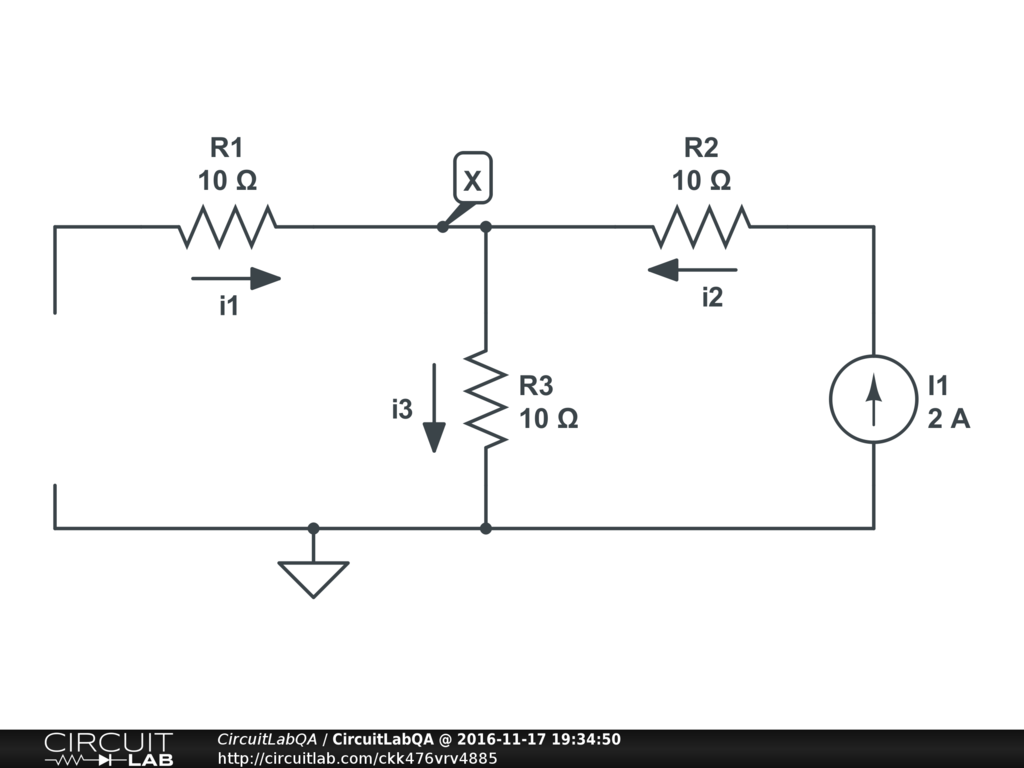## Superposition not working

 Question 1, what are the currents i1, i2, i3? Question 2, what is the voltage at node X? Solve using superposition.My problem is that I tried solving it using superposition and I get $I_1=6$, $I_2=2$, $I_3=8$, $V_X=80$. But the textbook (and the circuitlab simulation) both say the answer is different, $I_1=5$, $I_2=2$, $I_3=7$, $V_X=70$. What am I doing wrong? by dnadya November 17, 2016

 OK here's how you solve it using superposition. First only look at the voltage source. So get rid of current source I1 and solve everything, call this A:Just by looking at it you get $I_{1a}=I_{3a}=6$, $I_{2a}=0$, $V_{Xa}=60$. Now only look at the current source, so get rid of the voltage source V1 by making it short circuit (0 volts):Just by looking at this you get $I_{1b}=-1$, $I_{2b}=2$, $I_{3b}=1$, $V_{Xb}=10$. Now you add up the totals from the two sources: $$I_1 = I_{1a} + I_{1b} = 6 - 1 = 5$$ $$I_2 = I_{2a} + I_{2b} = 0 + 2 = 2$$ $$I_3 = I_{3a} + I_{3b} = 6 + 1 = 7$$ $$V_X = V_{Xa} + V_{Xb} = 60 + 10 = 70$$ That matches your textbook. Not sure what you did wrong but that's how to do it right. +2 votes by gavers7 November 17, 2016

 dnadya, I figured out what you were probably doing wrong by looking at @gavers7's answer. When you apply superposition, you have to "set the other sources to zero" -- but that has a different meaning for a current source and a voltage source. For a current source, it means $I=0$, zero current, i.e. an open circuit. So you can just remove the current source entirely. But for a voltage source it means $V=0$, which means a short circuit. So you can either leave the voltage source element in the circuit and set its voltage to 0, OR you can delete the voltage source and replace it with a wire. I think the mistake you made was to remove the voltage source V1 and just leave it open:When you do it this way you must have found $I_{1b}=0$, $I_{2b}=2$, $I_{3b}=2$, $V_{Xb}=20$. So then when you added things back together you found: $$I_1 = I_{1a} + I_{1b} = 6 + 0 = 6$$ $$I_2 = I_{2a} + I_{2b} = 0 + 2 = 2$$ $$I_3 = I_{3a} + I_{3b} = 6 + 2 = 8$$ $$V_X = V_{Xa} + V_{Xb} = 60 + 20 = 80$$ That matches what you (incorrectly!) found. Hope that helps! Remember: superposition = set sources to zero, which means different (almost opposite!) things for $V=0$ and $I=0$. +1 vote by mrobbins November 17, 2016

 firstly voltage source will be short circuit,,,and current source will be open circuit,,,,then follow the direction of current......,,,then add current,,,,,for i1,,,,,will 5,,cause,,,for S.C i"1=-1,, for O.C i'1=6,,,follow the step.i think you will be able to solve it +1 vote by MR_HIMEL December 06, 2016

CircuitLab's Q&A site is a FREE questions and answers forum for electronics and electrical engineering students, hobbyists, and professionals.

We encourage you to use our built-in schematic & simulation software to add more detail to your questions and answers.

Acceptable Questions:

• Concept or theory questions
• Practical engineering questions
• “Homework” questions
• Software/hardware intersection
• Best practices
• Design choices & component selection
• Troubleshooting

Unacceptable Questions:

• Non-English language content
• Non-question discussion
• Non-electronics questions
• Vendor-specific topics
• Pure software questions
• CircuitLab software support

Please respect that there are both seasoned experts and total newbies here: please be nice, be constructive, and be specific!